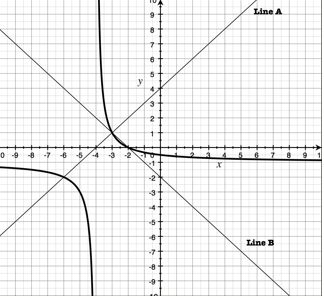## Monday, March 05, 2007

### Next Lesson: Rational FunctionsThe unit 5 test is over, vacation is over, and I'm ready to get back on track with posting.

In the last lesson, we started the Rational Functions unit (as I described in a previous post). As a warmup, I had students do some division work to explore what happens to a quotient as the divisor approaches zero. They did this visually (i.e. fitting smaller and smaller boxes into a fixed space) and numerically (filling in tables of values).

After they were clear on the effects of dividing by a number approaching 0, I gave them a graph with two linear functions on it, and asked them to work in teams to find the quotient function. They had to look at each value of x, estimate the y-values of the two lines, divide, and then plot a point for the quotient function. It doesn't sound like this would take too long, but I knew from experience that it would take at least a half hour (and it did!). But the division warmup did really help a lot, and my main goal was for them to really understand why a vertical asymptote occurs.

We then moved into some direct instruction where we reviewed the difference between 0/4 and 4/0, I introduced them to hyperbolas (the shape of the graph generated when you divide two linear functions... conic section definitions will have to wait), and we looked at vertical asymptotes and x-intercepts, and where they occur. Students have a lot of trouble with fractions (duh!) and this translates to confusion when trying to deal with rational functions. I hope that continued reminders about what happens when you divide by 0 will help them remember. Finally, I taught them the "as x approaches 2 from the left/right" type notation, with the minus/plus sign as superscript.

We did some example problems, and that was that. I came up with a good way of testing their understanding in the homework: I gave a graph of a hyperbola with two linear functions A and B, and asked them to determine which line was the numerator and which was the denominator.

In tomorrow's lesson, students will continue to practice these ideas, and I will introduce them to Rational Functions as a concept. We will solidify their understanding of x-intercepts, y-intercepts, and vertical asymptotes, and we will discuss the domain of rational functions. I will throw in some factoring, but nothing yet that simplifies (holes will be discussed a few lessons later on).